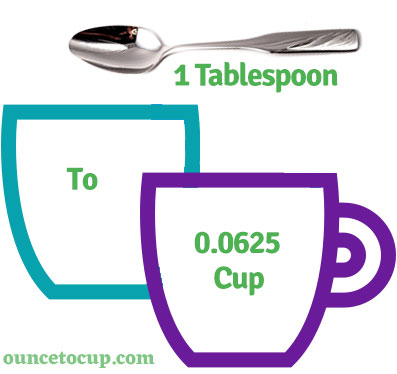# 4 Tablespoons to Cups (4 tbsp to c conversion)

Are you cooking your favorite dish? The detailed chart in the recipe includes the calculation of the 4 tablespoon to cup conversion.  Don't worry; use this calculator to determine how many 4 tablespoon equals cups in a minute.  This 4 tbsp to c converter gives an exact measurement for any recipe you prepare.

Tablespoon Value:

tbsp

Cup Value:

c

4 Tablespoon = 0.25 Cup
(4 tbsp = 0.25 c)

Try our auto 4 Tablespoon to Cup Calculator (Without Convert Button), Just change the first field value and you got final value.## How many cup is a 4 tbsp?

We know that the mass value of 4 tbsp is equal to 0.25 c. If you want to convert 4 tbsp to an equal number of cup, just divide the volume value by 16. Hence, 4 tablespoon is equal to 0.25 cup.

The Answer is: 4 US Tablespoons = 0.25 US Cup

4 tbsp = 0.25 cup

Many of them try to search or find an answer for what is 4 tablespoons in c? So, we’ll start with 4 tbsp to c conversion to know how big is 4 tbsp.

## How To Calculate 4 tbsp to cup?

To calculate 4 tablespoons to an equal number of cup, simply follow the steps below.

Tablespoons to Cups formula is:

Cup = Tablespoon / 16

Assume that we are finding out how many cup were found in 4 tbsp, divide by 16 to get the result.

Applying to Formula: cup = 4 tbsp / 16 = 0.25 cup.

## How To Convert 4 tbsp to cup?

• To convert 4 tablespoons to cup,
• Simply divide the tablespoon value by 16.
• Applying to the formula, cup = 4 tablespoons / 16 [4/16].
• Hence, 4 tablespoons is equal to 0.25 cup.
Tablespoons [tbsp]Cups [c]
1 tbps0.0625 cup
2 tbps0.125 cup
3 tbps0.1875 cup
4 tbps0.25 cup
5 tbps0.3125 cup
6 tbps0.375 cup
7 tbps0.4375 cup
8 tbps0.5 cup
9 tbps0.5625 cup
10 tbps0.625 cup
11 tbps0.6875 cup
12 tbps0.75 cup
13 tbps0.8125 cup
14 tbps0.875 cup
15 tbps0.9375 cup
16 tbps1 cup

## Reverse Calculation: How many tablespoons are in 4 cups?

• To convert 4 cups to tbsp,
• Simply multiply the 4 cup by 16.
• Then, applying the formula, tbsp = 4 cup * 16 [4x16 = 64].
• Hence, 4 cup is equal to 64 tablespoon.

### Related Converter:

Formula: tablespoon to cup

cup = tablespoon / 16

Applying to Formula,

c = 4/16 = 0.25

4 tablespoon = 0.25 cup